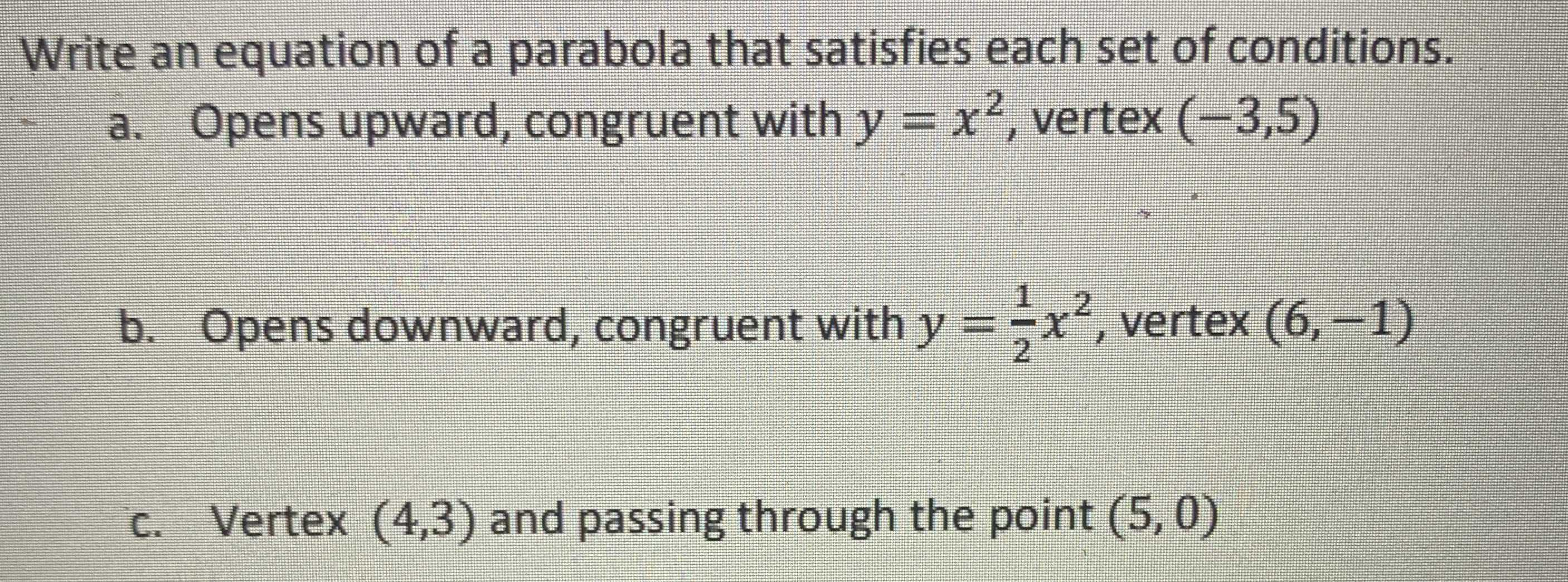### Still have math questions?

Algebra
QuestionWrite an equation of a parabola that satisfies each set of conditions.

a. Opens upward, congruent with $$y = x ^ { 2 }$$ , vertex $$( - 3,5 )$$

b. Opens downward, congruent with $$y = \frac { 1 } { 2 } x ^ { 2 }$$ , vertex $$( 6 , - 1 )$$

$$a.y= (x+ 3)^{2} + 5$$
$$b.y= \frac{1}{2} (x- 6)^{2} - 1$$